# Points to Remember: Chemical Bonding Notes | Study Inorganic Chemistry - Chemistry

## Chemistry: Points to Remember: Chemical Bonding Notes | Study Inorganic Chemistry - Chemistry

The document Points to Remember: Chemical Bonding Notes | Study Inorganic Chemistry - Chemistry is a part of the Chemistry Course Inorganic Chemistry.
All you need of Chemistry at this link: Chemistry

Points to be Remembered

1. Determination of Hybridization and Shape of a Molecule: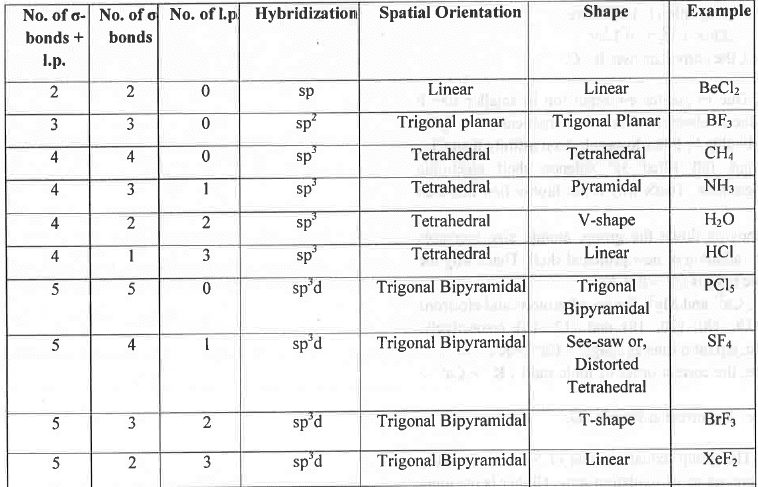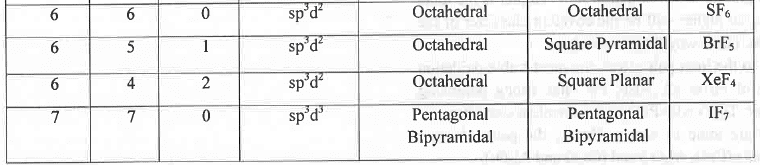A. Determine the Shape and Hybridization of BrF3:
The valence shell electronic configuration of Br atom is: 5s25p55d°.
Br atom in ground state: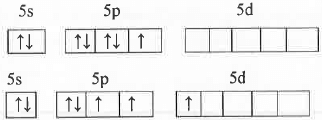Br atom in BrF3 molecule: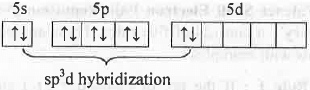The hybrid orbitals used by bromine atom in BrF3 are sp3d. Hence, its spatial orientation is Trigonal Bipyramidal. But, due to the presence of two l.p. of electrons, its shape will be T-shape.
B. Determine the Shape and Hybridization of XeO2F2:
The valence shell electronic configuration of Xe atom is : 5s25p65d°.
Xe atom in ground state: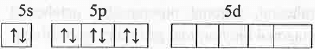Xe atom in excited state: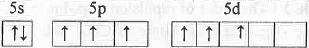Xe atom in XeO2Fmolecule: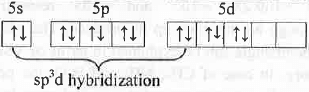Hence, Xe atom in XeO2F2 is sp3d hybridized. Hence, it has TBP spatial orientation. But, due to the presence of one l.p. of electrons it will have distorted Td shape.
C. Determine the Shape and Hybridization of Br3+:
The valence shell electronic configuration of Br+ ion is : 4s24p44d°.
Br+ in ground state: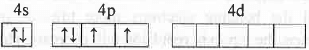Br+ in excited state: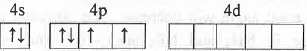Br+ in Br3+ ion: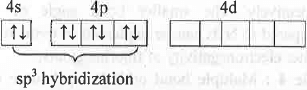Hence, Br3+ has sp3 hybridization and its geometry of is tetrahedral. But, due to presence of two lone pairs of electrons, it has V-shape.
The valence shell electronic configuration of I" ion is: 5s25p65d°.
I- in ground state: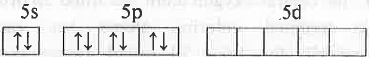F in excited state: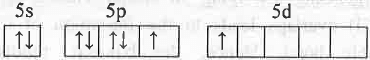F in l3- ion: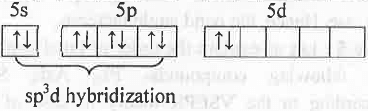Hence, I3- has sp3d hybridization. Hence, it has trigonal bipyramidal spatial orientation. But, due to presence of three lone pairs of electrons, it has linear shape.
2. Valence Shell Electron Pair Repulsion (VSEPR)
Theory: It consists of five rules. These are explained below with examples:

• Rule 1: If the no. of (σ-bond + 1.p.) around a central atom are 2, 3, 4, 5, 6 and 7 respectively then the geometry of the molecule will be Linear, Trigonal Planar, Tetrahedral, Trigonal Bi- pyramidal, Octahedral and Pentagonal Bi- pyramidal respectively. Hence, BeCl2, BF3, CH4, PC15, SF6 and IF7 has linear, trigonal planar, tetrahedral, trigonal bipyramidal, octahedral and pentagonal bipyramidal geometry respectively
• Rule 2: The order of repulsion : 1.p.-1.p. > 1.p.-b.p. > b.p.-b.p. The bond angle of CH4, NH3 and H20 are ~ 109°28',~107° and ~105° respectively although all these are sp3 hybridized. This change in bond angle can be explained in terms of VSEPR theory. In case of CH4, NH3 and H2O, the no. of lone pairs presents around the central atom is 0, 1 and 2 respectively. Hence, the l.p.-l.p. repulsion will decrease the bond angle in H2O most while CH4 will have the ideal bond angle 109°28' due to absence of lone pair.
• Rule 3: If the terminal atoms become more electronegative than the central atom, these will pull the bonding electrons more towards itself. Hence, the b.p.-b.p. repulsion will decrease. Hence, the bonds will come closer to each other. Hence, the bond angle will decrease. The observed bond angle in NH3 and NF3 are — 107° and ~102° respectively. The smaller bond angle of NF3 compared to NH3 can be explained in terms of the higher electronegativity of fluorine atoms.
• Rule 4: Multiple bond orbitals repel more than single bond orbitals. The observed bond angle in OF2 and OCl2 are ~ 103° and ~ 111 ° respectively. This can be explained by VSEPR theory. In case of OCl2, the central oxygen atom has filled 2p orbital while terminal chlorine atoms has vacant energetically low lying 3d orbital. Hence, pπ(O)- dπ(Cl) overlaps leads to the formation of Cl-O double bond. Hence, the b.p.-b.p. repulsion increase. Hence, the bond angle increase.
• Rule 5: Let us explain the order of bond angle of the following compounds- PI3, Asl3, Sbl3. According to the VSEPR theory in case of the incomplete valence shell the contraction in bond angle due to the l.p.-l.p. or l.p.-b.p. is more significant than that for complete valence shell. Hence, all these compounds has X-M-X bond angle much less than 109°. As the size of the central atom increase and electronegativity decrease, the b.p.- b.p. repulsion will decrease and hence, bond angle will decrease. Hence, SbX3 compounds will have least X-M-X bond angle.

3. Covalency in a Molecule (Fajan's Rule): When a cation come closer to an anion, the cation will try to deform the anion and vice versa. But, due to the much smaller size of a cation, it will deform the anion much more than that does by anion on cation. Such kind of deformation in a molecule is known as Polarisation. The power with which a cation polarize an anion is known as polarising power of cation and the ability of an anion to become polarize by the cation is known polarisability of anion.
According to Fajan higher is the positive charge on cation and smaller is the size of the cation, greater will be the polarising power of cation and hence, higher will be the covalent character of the molecule. The polarising power which ia termed as ionic potential (ϕ) of a cation is defined as the charge of cation to the size of the cation i.e. ϕ = charge of cation/size of cation. Hence, the polarising power of the following cations are : Al3+ > Mg2+ > Na+.
Also, according to Fajan higher is the negative charge on anion and larger is the size of the anion, greater will be the polarisability of anion and hence, higher will be the covalent character of the molecule. Hence, the polarisability of the following anions are : I- > Br- > Cl- > F-.
Application of Fajan's Rule:

• Hydration of a Salt: Greater is the covalent character of a molecule, higher will be the chance of hydration. Hence, higher is the polarising power of cation and greater is the polarisability of anion, more will be the chance of hydration of the salt. Hence, the order of hydration of the following salts are : AlCl3 > MgCl2 > NaCl.
• Hydrolysis of a Salt: Greater is the covalent character of a molecule, higher will be the chance of hydrolysis. Hence, higher is the polarising power of cation and greater is the polarisability of anion, more will be the chance of hydrolysis of the salt. Hence, the order of hydrolysis of the following salts are : AlCl3 > MgCl2 > NaCl.
• Thermal Stability of Salts: Greater is the ionic potential of a cation, smaller will be the temperature at which it will decompose i.e. lower will be the thermal stability. Hence, greater is the covalent character of a molecule, lower will be the thermal stability of the molecule. Hence, the order of the thermal stability of the following compounds are: BeC03 < MgCO3 < CaCO3.
• Basicity of Oxides: Basicity of an oxide depends on the ability to donate its lone pair of electrons to the other species (Lewis Acid). Greater is the electron donation, higher will be basicity of the oxide. Now, greater is the ionic potential of the cation, more it will pull the lone pair of electrons from oxygen atom making the lone pair of electrons less available for donation to other species (Lewis Acid). Hence, the basicity will decrease. Hence, the order of the basicity of the following oxides are: Na20 < MgO < Al2O3.
• Solubility: 'Like dissolves like' is the thumb rule for the dissolution of a solute in a solvent. Greater is the covalent character of a molecule, more it will become soluble in organic solvent like alcohol. But, higher is the ionic character, more it will become soluble in polar solvent like water. That's why KI is more soluble in alcohol than KCl.
• Colour of Some Salts: SnO2 is colourless while SnS2 is coloured. This can be explained in terms of Fajan's rule. In both cases Sn is present as Sn4+ while the anions are O2- and S2-. Due to the smaller size and higher positive charge on Sn, Sn4+ has very high ionic potential. Due to larger size of S2-, it has high polarisability. Hence, the energy required for electronic transition from S2- to Sn4+ is less and falls in the visible region. But, due to lower polarisability of O2- the energy required for electronic transition from O2- to Sn4+ is very high and falls in the UV region. That's why SnS2 become coloured while SnO2 become colourless.
•  Instability of Some Salts: Some compounds like Pbl4 is almost non-existent. This can be explained by Fajan's rule. The ionic potential of Pb4+ is very high due to its smaller size and very high positive charge. Hence, it will induce highly polarisable I' for electronic transition from two f to Pb4+ converting Pb4+ into Pb2+. As a result Pblis form. Pbl4 = Pbl2 + I2

4. Born Haber Cycle: The Born-Haber Cycle for the formation of NaCl crystal from its constituent elements is as follows: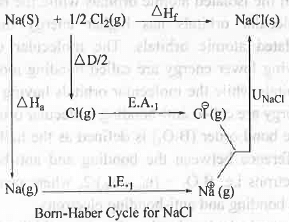Where, ΔHa, ΔD/2, ΔHf, I.E.1 , E.A.1, UNacl terms represent the heat of atomization of metallic sodium, half of the bond dissociation energy of chlorine molecule , enthalpy of formation of NaCl , first ionization energy of sodium, first electron affinity of chlorine and Lattice energy of NaCl.
Hence, from the Born-Haber Cycle it is clear that no electronegativity term is involved in the equation. Hence, electronegativity cannot be estimated by using Born-Haber Cycle in case of NaCl.

5. Lattice Energy: Lattice energy has an important role in determining the melting point of a compound. Greater is the lattice energy of a compound, higher will be the melting point of the compound. Lattice Energy (U) is given by Bom-Lande equation: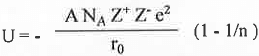Where, A = Madelung constant, n = Born exponent, NA = Avogadros no., r0 = Equilibrium inter-nuclear distance, Z+Z- is the product of opposite charges. Now let us consider the melting points o f Al2O3, CaO, NaF, KCl.
The various ions are Al3+, O2-, Ca2+, Na+, F- and Cl-. Hence, Al2O3 will have highest lattice energy due to its highest Z+Z- value. Then, due to higher Z+Z- value, CaO will have second highest lattice energy. Chloride ion is larger than the fluoride ion and potassium ion is larger than the sodium ion . Hence, NaF will have higher lattice energy than the KCl. Hence, the decreasing order of lattice energy is : Al2O3 > CaO > NaF > KCI.
6. Molecular Orbital Theory (M.O.T.):

• If n no. of atomic orbitals of one atom combine with m no. of atomic orbitals of another atom, (m+n) no. of molecular orbitals will be formed. Half of the molecular orbitals has lower energy than the isolated atomic orbitals while the rest half molecular orbitals has higher energy than the isolated atomic orbitals. The molecular orbitals having lower energy are called bonding molecular orbitals while the molecular orbitals having higher energy are called anti-bonding molecular orbitals.
• The bond order (B.O.) is defined as the half of the difference between the bonding and anti-bonding electrons i.e. B.O. = (nb - nab)/2, where nb and nab, are bonding and anti-bonding electrons.
• Greater is the bond order of a species higher will be its stability. Hence, greater energy will require to break the bond leading to high bond dissociation energy.
• Addition of electrons to bonding orbital or, removal of electrons from anti-bonding orbital increase the bond order making the system more stable.
• Addition of electrons to anti-bonding orbital or, removal of electrons from bonding orbital decrease the bond order making the system less stable.
The document Points to Remember: Chemical Bonding Notes | Study Inorganic Chemistry - Chemistry is a part of the Chemistry Course Inorganic Chemistry.
All you need of Chemistry at this link: ChemistryUse Code STAYHOME200 and get INR 200 additional OFF

## Inorganic Chemistry

49 videos|71 docs|16 tests

Track your progress, build streaks, highlight & save important lessons and more!

,

,

,

,

,

,

,

,

,

,

,

,

,

,

,

,

,

,

,

,

,

;